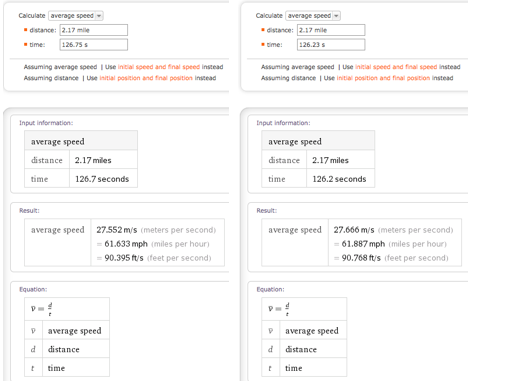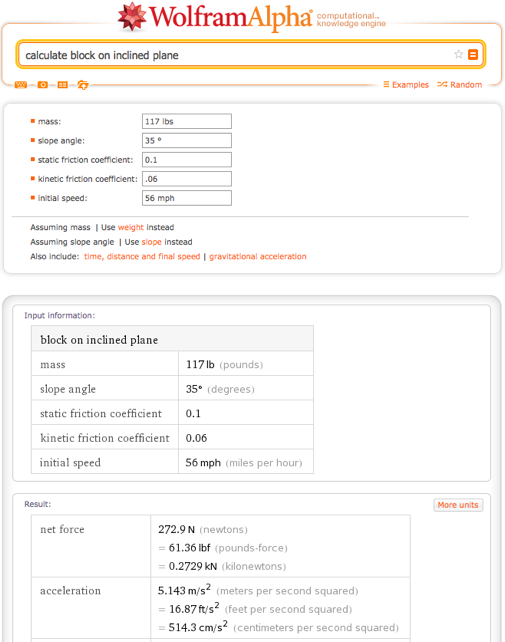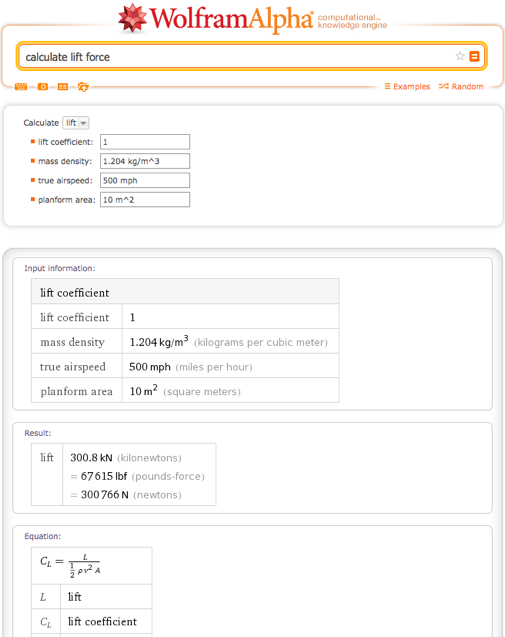# Computing for the Gold: Exploring Olympic Science with Wolfram|Alpha

February 17, 2014 —Comments Off

The start of the XXII Olympic Winter Games means one thing for me: at least six hours a day of watching people ski down treacherous slopes, do crazy 720-degree spins with their snowboards, and perform triple toe loop jumps. Whether or not you’re spending every waking moment watching athletically superior individuals accomplish seemingly impossible feats, you can take this opportunity to explore some of Wolfram|Alpha’s math and physics calculators. Now Wolfram|Alpha can add a fun science and math spin to the Olympics.

Let’s start with alpine skiing. The following is a simple math problem, and we use one of Wolfram|Alpha’s many math calculators. In a surprising turn of events, Austrian Matthias Mayer won gold with a 2-minute-6.23-second run down Rosa Khutor, the 2.17-mile course. On the other hand, America’s favorite for gold, Bode Miller, finished 8th with a 2-minute-6.75-second run. We can use Wolfram|Alpha to compare Mayer’s and Miller’s average speeds. In fact, when you query Wolfram|Alpha for average speed, you are getting an online calculator.We can also use a physics calculator to help us understand the Olympics. For example, the inclined plane problem from physics can be thought of as an extreme simplification of the inrun portion (that’s the downhill part) of ski jumping. Wolfram|Alpha can calculate the net force, acceleration, and much more for your inclined plane problems with just a few inputs. In this example, we will use statistics from Kamil Stoch, the gold medalist for the normal ski jump course, and the RusSki Gorki course in Sochi. Keeping in mind that snow and ice have fairly low friction coefficients, we’ll use small estimates for those:After the inrun portion comes the jumping (or as I like to call it, flying) section of ski jump. We can use Wolfram|Alpha’s drag force calculator to compute the drag force exerted on Kamil Stoch right after his takeoff. Using a drag coefficient of .6 and assuming Stoch’s shoulders are 2-feet wide, Wolfram|Alpha calculates an approximate drag force.A ski jumper wants to minimize his (or her, starting with this year’s games) drag force and maximize the lift force to be able to land as far as possible. Wolfram|Alpha also has a lift force calculator that takes in various different types of inputs and gives different results.But that’s enough about skiing for now. Let’s move onto my personal favorite: figure skating events. Wolfram|Alpha has calculators to help you understand figure skating concepts like angular momentum. For example, during a scratch spin, the 15-year-old Yulia Lipnitskaia would start with her arms and one leg extended and then slowly bring them in to increase her speed. This is a demonstration of conservation of angular momentum. So let’s use Wolfram|Alpha’s moment of inertia calculator and angular momentum calculator to see exactly how much faster she can go by bringing in her arms. Start by calculating Yulia’s moment of inertia (for simplicity’s sake, we approximate her as a cylinder) when her arms and legs are pulled into her body.Now assuming her leg and two arms weigh about 20 pounds, first calculate the moment of inertia for her torso plus a leg, then add the moment of inertia for her two arms and other leg. Moment of inertia for her torso:Moment of inertia for each arm:Moment of inertia for her leg:So her total moment of inertia for when her arms and leg are extended is 4.07 kgm^2. Now, by the conservation of angular momentum, we can find the difference in the angular velocity of Yulia between the beginning of her scratch spin and the end. Suppose she starts out going at 6 radians per second.After calculating the angular momentum, you can change the drop-down menu to calculate the angular velocity using 24.42 Js as angular momentum and .40 kgm^2 as the moment of inertia from earlier calculations.Now she’s rotating almost 10 times faster than she was by simply pulling her arms and legs in! So during commercial breaks, you can use one of Wolfram|Alpha’s calculators to gain a better understanding of the science behind the events. There are many more math and science calculators available, so check them out in between cheering “Go team USA!” And don’t forget, you can use Wolfram|Alpha to track team USA’s progress during the Sochi Olympics. For more information on that, take a look at Allison Taylor’s blog post.